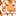# App Development

Announcements
Read about the latest Qlik Community enhancements on the Community News blog!
cancel
Showing results for
Did you mean:Contributor III

## How to keep fixed color a one of the bar in bar chart

HI Team,

I have a bar chart where I am showing comparison between two values. one bar will be with a fixed value and the other will get changed when applies filters.

I want to keep one of the bar with a fixed color in bar chart, but when I filter,both the bars(bar with Fixed value and actual value) are getting changed.

Attached is my QVf file.

Thanks,

Murali

5 RepliesContributor III
Author

Hi,

Below is the expression I have Used.

Measure M1= aggr(sum(claimAmount),cost)/sum(aggr(MM,cost,memId,PlanId)

Measure M2=aggr(sum(claimAmount1),cost)/sum(aggr(MM1,cost,memId1,PlanId1))

For M1 bar color should be Fixed, M2 bar color should be vary on applying filters. For this I have given custom expression as below at bar charts Colors and Legent>colors>Cusotom Expression.

if(aggr(sum(claimAmount),cost)/sum(aggr(MM,cost,memId,PlanId)) >(aggr(sum(claimAmount1),cost)/sum(aggr(MM1,cost,memId1,PlanId1)))*60/100, 'green',

if(aggr(sum(claimAmount),cost)/sum(aggr(MM,cost,memId,PlanId)) <(aggr(sum(claimAmount1),cost)/sum(aggr(MM1,cost,memId1,PlanId1)))*60/100, 'yellow',

if(aggr(sum(claimAmount),cost)/sum(aggr(MM,cost,memId,PlanId)) >(aggr(sum(claimAmount1),cost)/sum(aggr(MM1,cost,memId1,PlanId1)))*50/100, 'yellow',

red())))Thanks,

MuraliContributor III
Author

Hi,

Below is the new ticket with more info in ticket and attached qvf file. url

How to keep fixed color a one of the bar in bar chartContributor III
Author

Hi,

Can any one please for the help on this requirement.

Thanks,

MuraliPartner

Hi Murali,

Once you have created, please go to the dimension and click on edit,

You will have two tabs, 1 for Edit dimension and 1 for value colours.

Inside the Value colors tab, you can set the color for specific dimension values.

Using Colors with Qlik Sense Visualizations, Measures and DimensionsContributor III
Author

Hi,

If I create like that then the color will be effected for both the bars. I want one bar with Fixed color and other varies by value selected on filter.

Below is my requirement.

Measure M1= aggr(sum(claimAmount),cost)/sum(aggr(MM,cost,memId,PlanId)

Measure M2=aggr(sum(claimAmount1),cost)/sum(aggr(MM1,cost,memId1,PlanId1))

For M1 bar color should be Fixed, M2 bar color should be vary on applying filters. For this I have given custom expression as below at bar charts Colors and Legent>colors>Cusotom Expression.

if(aggr(sum(claimAmount),cost)/sum(aggr(MM,cost,memId,PlanId)) >(aggr(sum(claimAmount1),cost)/sum(aggr(MM1,cost,memId1,PlanId1)))*60/100, 'green',

if(aggr(sum(claimAmount),cost)/sum(aggr(MM,cost,memId,PlanId)) <(aggr(sum(claimAmount1),cost)/sum(aggr(MM1,cost,memId1,PlanId1)))*60/100, 'yellow',

if(aggr(sum(claimAmount),cost)/sum(aggr(MM,cost,memId,PlanId)) >(aggr(sum(claimAmount1),cost)/sum(aggr(MM1,cost,memId1,PlanId1)))*50/100, 'yellow',

red())))Tags
Community Browser